Next: Degenerate Electron Gases Up: Three-Dimensional Quantum Mechanics Previous: Fundamental Concepts

# Particle in a Box

Consider a particle of masstrapped inside a cubic box of dimension(see Sect. 5.2). The particle's stationary wavefunction,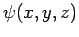, satisfies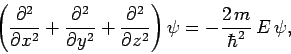(492)

whereis the particle energy. The wavefunction satisfies the boundary condition that it must be zero at the edges of the box.

Let us search for a separable solution to the above equation of the form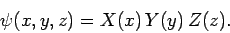(493)

The factors of the wavefunction satisfy the boundary conditions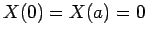,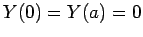, and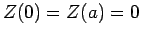. Substituting (493) into Eq. (492), and rearranging, we obtain(494)

where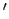denotes a derivative with respect to argument. It is evident that the only way in which the above equation can be satisfied at all points within the box is if(495)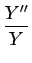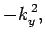(496)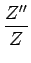(497)

where,, and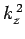are spatial constants. Note that the right-hand sides of the above equations must contain negative, rather than positive, spatial constants, because it would not otherwise be possible to satisfy the boundary conditions. The solutions to the above equations which are properly normalized, and satisfy the boundary conditions, are [see Eq. (310)]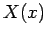(498)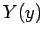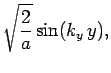(499)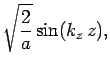(500)

where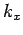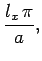(501)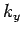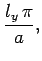(502)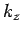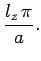(503)

Here,,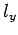, andare positive integers. Thus, from Eqs. (494)-(497), the energy is written [see Eq. (308)]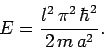(504)

where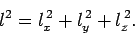(505)Next: Degenerate Electron Gases Up: Three-Dimensional Quantum Mechanics Previous: Fundamental Concepts
Richard Fitzpatrick 2010-07-20